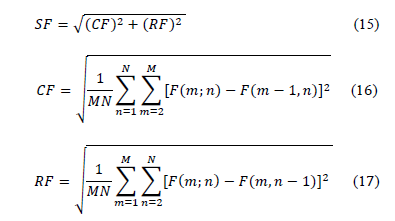# Calculation of spatial frequency

Hello ITK,
i want to compute “spatial frequency” for wavelet. In these publication (DOI: 10.17694/bajece.24838,H. Boztoprak ) they define it as:with M, N the voxel indice and F his value.
So i create a code like this which is suboptimal.

``````def SpatialFrequency(image):
SizeMatrix=image.GetSize()
Square_diff_x=0
Square_diff_y=0
Square_diff_z=0
Nvoxel=0
for x in range(SizeMatrix-1):
for y in range(SizeMatrix-1):
for z in range(SizeMatrix-1):
Square_diff_x=Square_diff_x+(image.GetPixel(x+1,y,z)-image.GetPixel(x,y,z))**2
Square_diff_y=Square_diff_y+(image.GetPixel(x,y+1,z)-image.GetPixel(x,y,z))**2
Square_diff_z=Square_diff_z+(image.GetPixel(x,y,z+1)-image.GetPixel(x,y,z))**2
Nvoxel=Nvoxel+1
SF=(Square_diff_x/(Nvoxel)+Square_diff_y/(Nvoxel)+Square_diff_z/(Nvoxel))**0.5
return SF

``````

can you help me to improve it?
Thank you,
Cyril Jaudet

this new code is really faster but not give the same result. Any clue?

``````def SpatialFrequencyOptim(image):
dimension = 3
offset =(1,0,0) # offset can be any vector-like data
translation_dx = sitk.TranslationTransform(dimension, offset)
image_dx=reechantillonage(image,translation_dx,0)
offset =(0,1,0) # offset can be any vector-like data
translation_dy = sitk.TranslationTransform(dimension, offset)
image_dy=reechantillonage(image,translation_dy,0)
offset =(0,0,1) # offset can be any vector-like data
translation_dz = sitk.TranslationTransform(dimension, offset)
image_dz=reechantillonage(image,translation_dz,0)
imageSF=(image-image_dx)**2+(image-image_dy)**2+(image-image_dz)**2
imageSF=sitk.SumProjection(imageSF,2)
imageSF=sitk.SumProjection(imageSF,1)
imageSF=sitk.SumProjection(imageSF,0)
#SF=imageSF.GetPixel(0,0,0)**0.5 #for testing
SF=(imageSF.GetPixel(0,0,0)/(image.GetSize()*image.GetSize()*image.GetSize()))**0.5
return SF
``````

Hi! I don’t think I can, not familiar with those `sitk` functions, or `reechantillonage`.

@drcjaudet Are you developing those functions for yourself or getting them from elsewhere?
@blowekamp might have a better idea about if you are using correctly those `sitk` functions.

Hello @drcjaudet

Not sure about your code, as the function `reechantillonage` is not shown. I suspect that it is a call to resample with zero padding. You should be able to do this via numpy (we don’t want to enforce the spatial overlap between images). I believe the code below does what you want for an arbitrary dimensional image (haven’t tested it so be cautious):

``````import SimpleITK as sitk
import numpy as np

def SpatialFrequencyOptim(image):
dim = image.GetDimension()
size = image.GetSize()
#work in numpy because we don't want to enforce spatial overlap
npa = sitk.GetArrayViewFromImage(image)
sq_diff = 0.0
for i in range(dim): #iterate over all image dimensions
slc1 = [slice(None)]*dim
slc1[i] = slice(0,size[i]-1)
slc2 = [slice(None)]*dim
slc2[i] = slice(1,size[i])
sq_diff+= np.sum((npa[tuple(slc2)] - npa[tuple(slc1)])**2)
return sq_diff/np.prod(size)

dim=3
img = sitk.GaussianSource(outputPixelType=sitk.sitkUInt8, size=*dim, sigma=*dim, mean=*dim)
sitk.Show(img)

res = SpatialFrequencyOptim(img)
``````
2 Likes

Thank a lot for the tips and code.
There was a problem with my image since npa.shape=(432L, 288L, 288L) was different than size(288,288,432); It was resolved with the following code were i just pass the an np matrix.

``````def SpatialFrequencyOptim2(matrix):
sq_diff = 0.0
size=matrix.shape
dim=len(size)
for i in range(dim): #iterate over all image dimensions
slc1 = [slice(None)]*dim
slc1[i] = slice(0,size[i]-1)
slc2 = [slice(None)]*dim
slc2[i] = slice(1,size[i])
sq_diff+= np.sum((matrix[tuple(slc2)]- matrix[tuple(slc1)])**2)
return sq_diff/np.prod(size)
``````

the different time calculation were:
solution 1, time=60.38s
solution 2, time=1.96 secondes
solution 3, Zivy one, time=1.87 secondes
thank a lot)

2 Likes